Learn all Concepts of Chapter 4 Class 10 (with VIDEOS). Check - Quadratic Equations - Class 10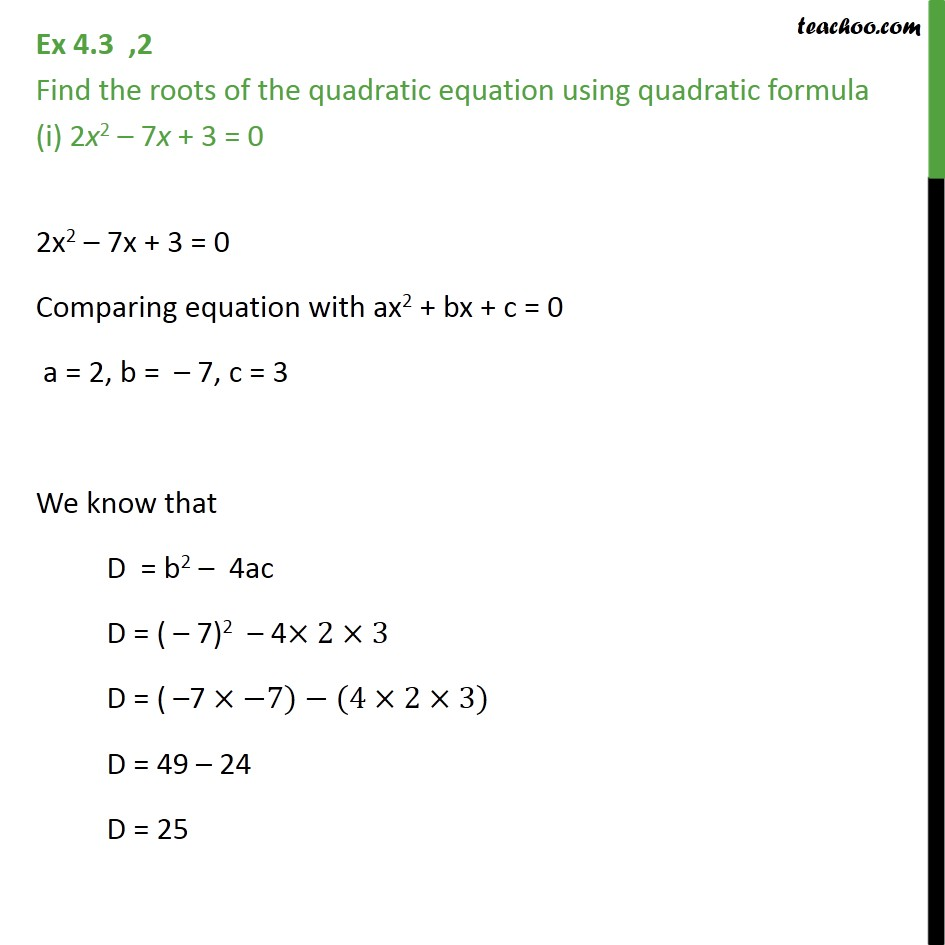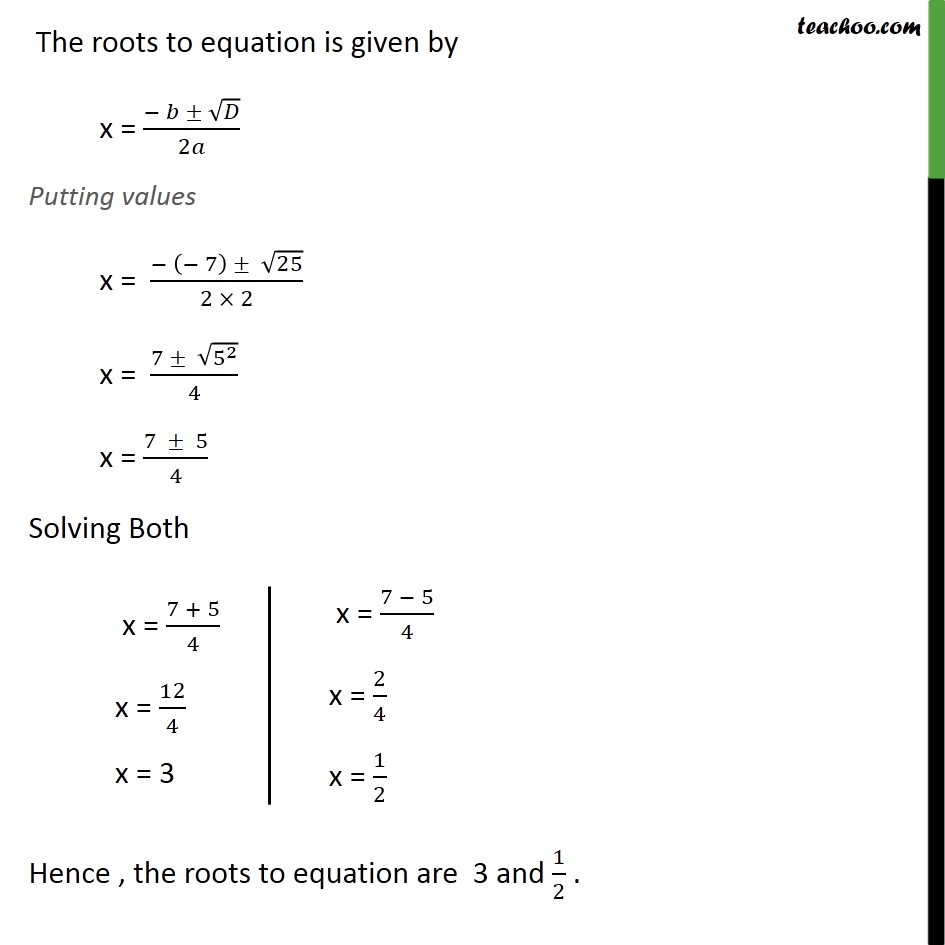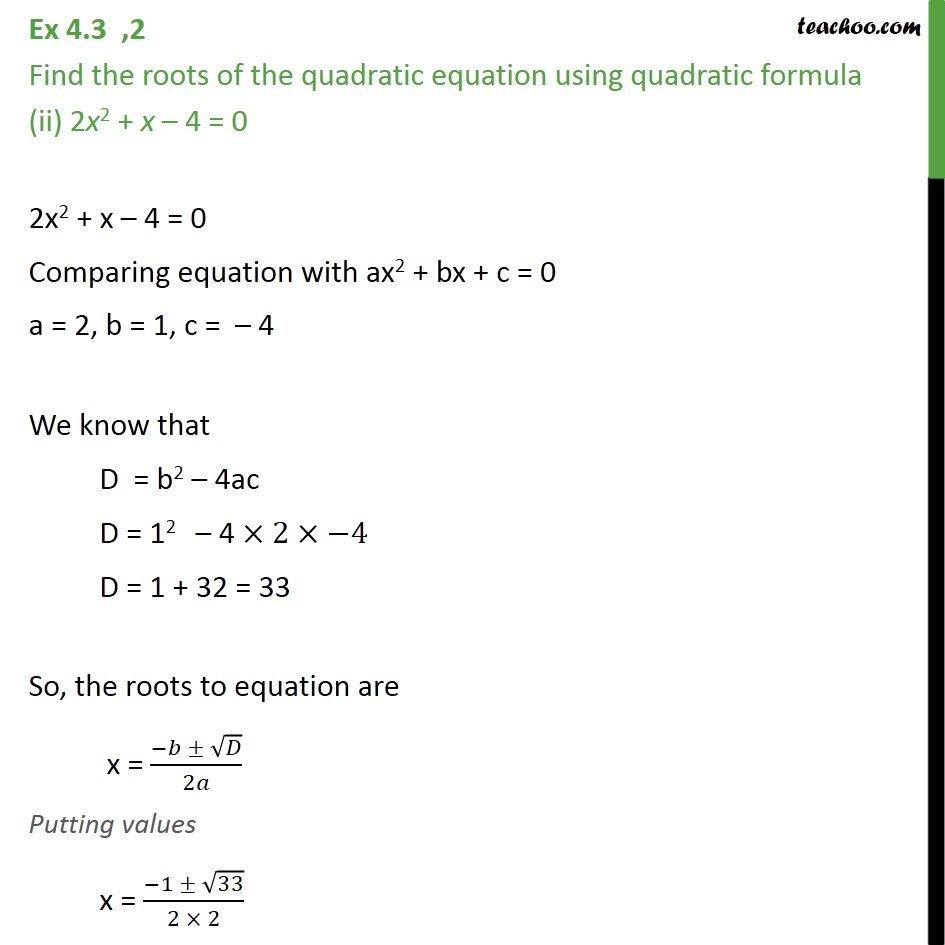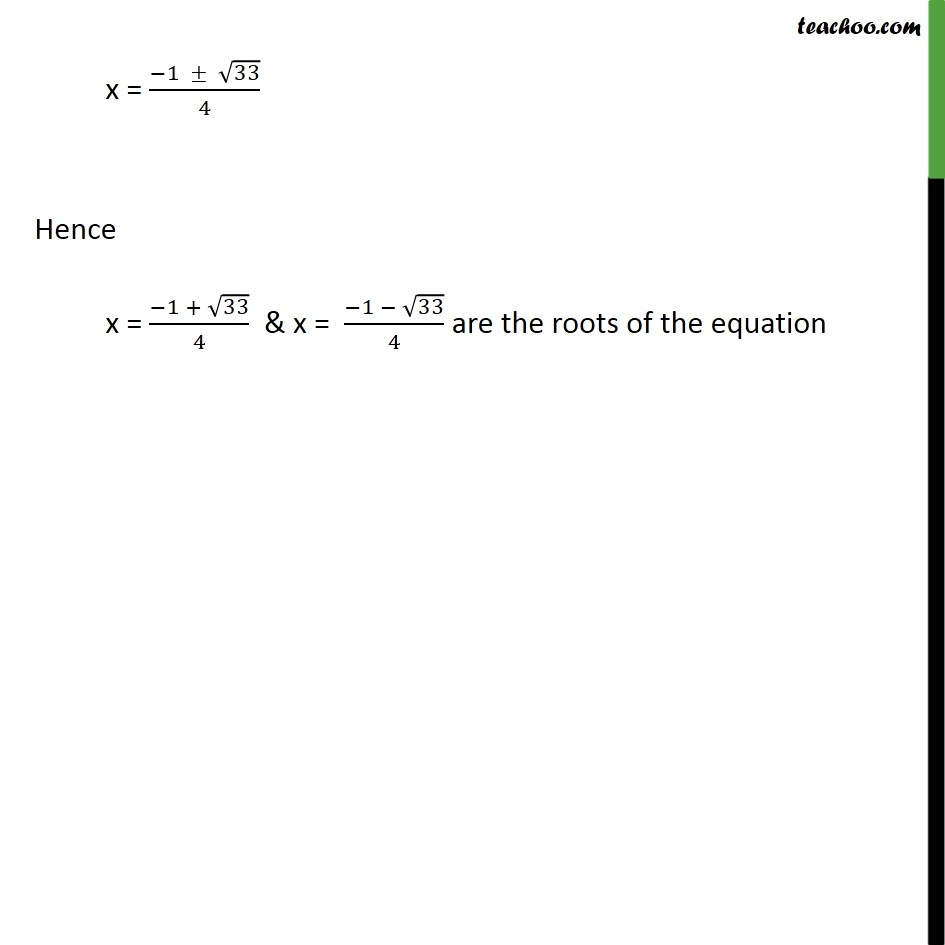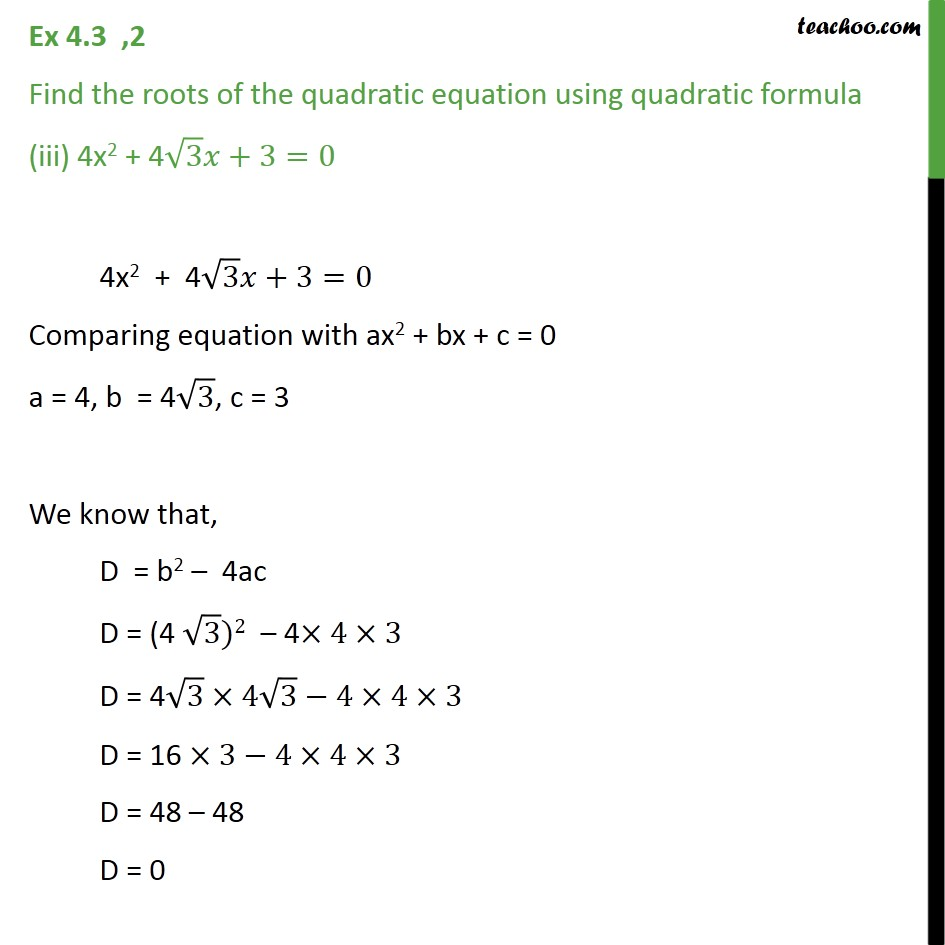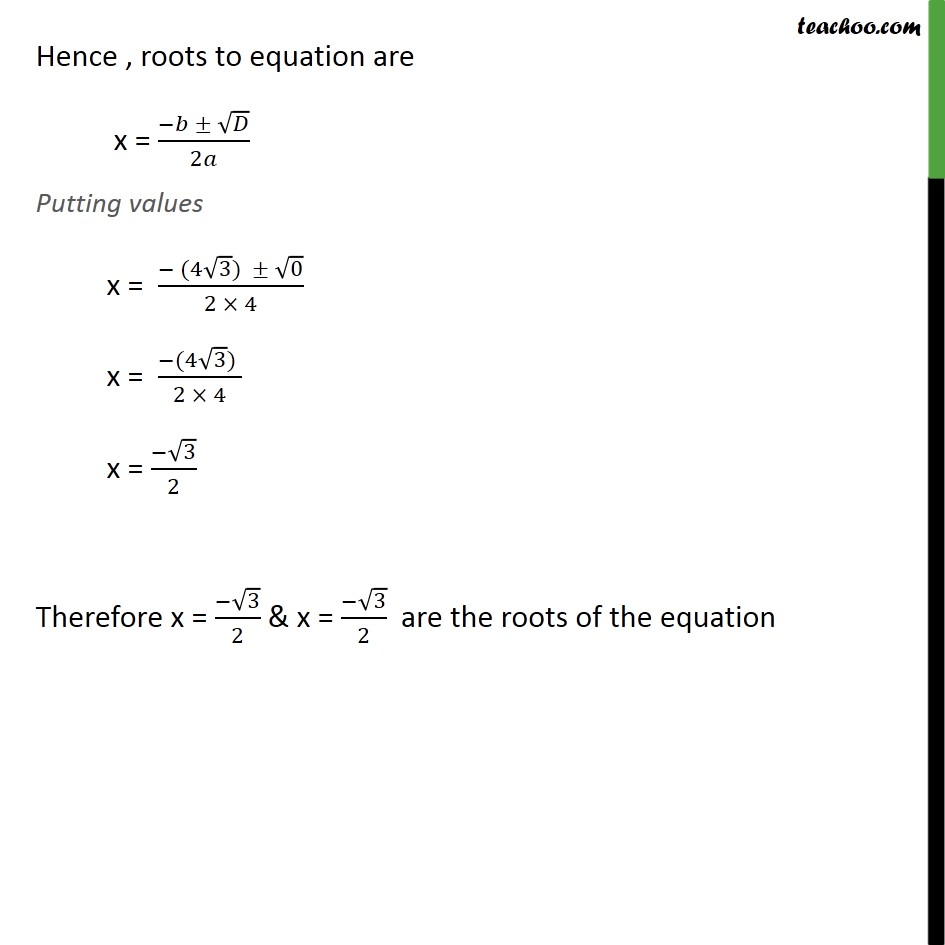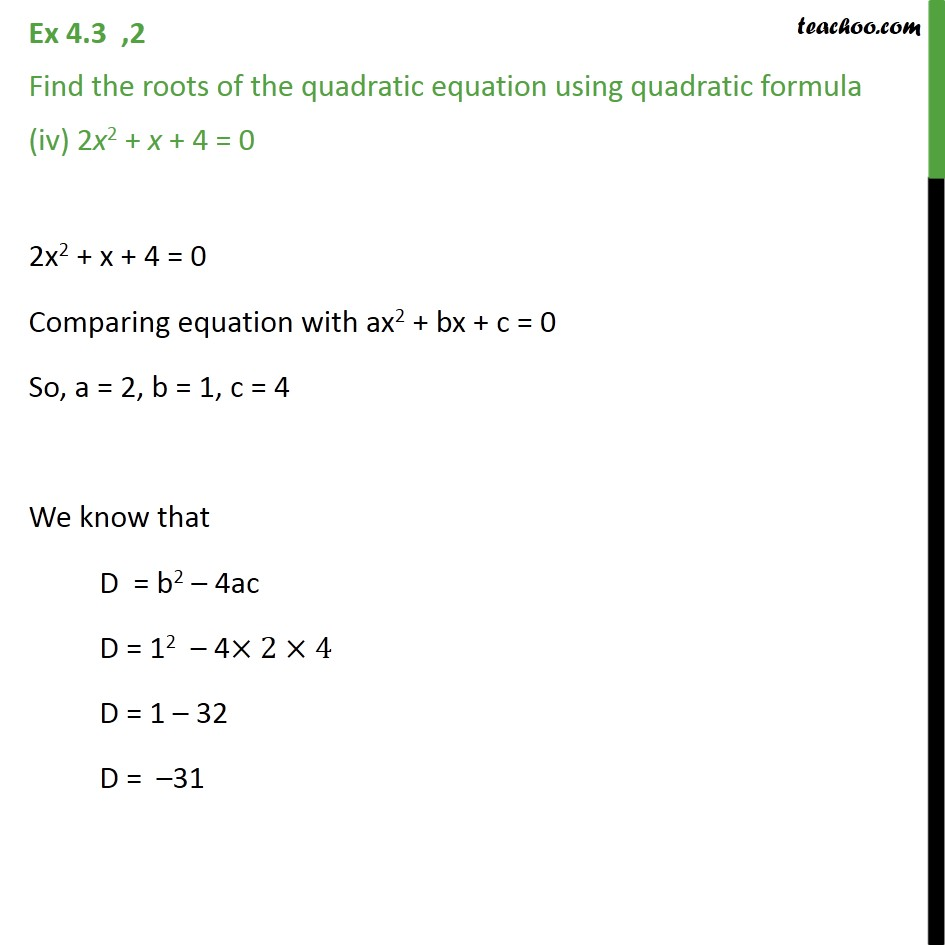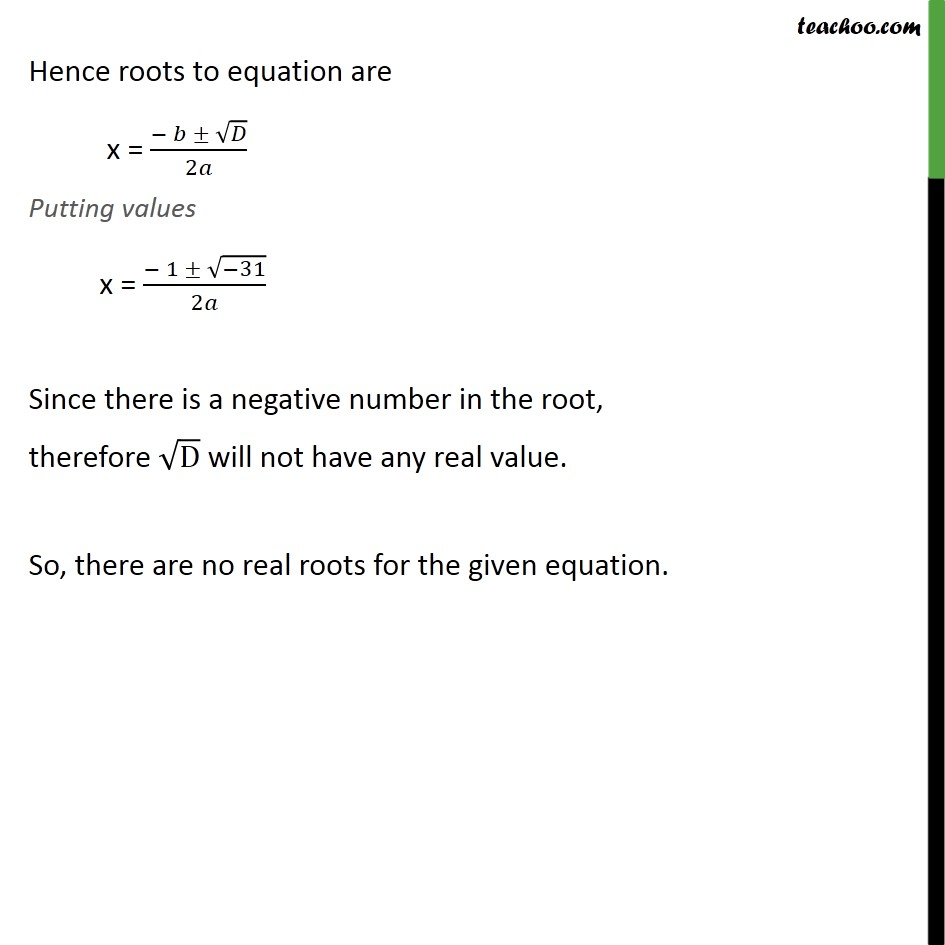1. Chapter 4 Class 10 Quadratic Equations
2. Serial order wise
3. Ex 4.3

Transcript

Ex 4.3 ,2 Find the roots of the quadratic equation using quadratic formula (i) 2x2 7x + 3 = 0 2x2 7x + 3 = 0 Comparing equation with ax2 + bx + c = 0 a = 2, b = 7, c = 3 We know that D = b2 4ac D = ( 7)2 4 2 3 D = ( 7 7) (4 2 3) D = 49 24 D = 25 The roots to equation is given by x = ( )/2 Putting values x = ( ( 7) 25)/(2 2) x = (7 (5^2 ))/4 x = (7 5)/4 Solving Both Hence , the roots to equation are 3 and 1/2 . Ex 4.3 ,2 Find the roots of the quadratic equation using quadratic formula (ii) 2x2 + x 4 = 0 2x2 + x 4 = 0 Comparing equation with ax2 + bx + c = 0 a = 2, b = 1, c = 4 We know that D = b2 4ac D = 12 4 2 4 D = 1 + 32 = 33 So, the roots to equation are x = ( )/2 Putting values x = ( 1 33)/(2 2) x = ( 1 33)/4 Hence x = ( 1 + 33)/4 & x = ( 1 33)/4 are the roots of the equation Ex 4.3 ,2 Find the roots of the quadratic equation using quadratic formula (iii) 4x2 + 4 3 +3=0 4x2 + 4 3 +3=0 Comparing equation with ax2 + bx + c = 0 a = 4, b = 4 3, c = 3 We know that, D = b2 4ac D = (4 3)2 4 4 3 D = 4 3 4 3 4 4 3 D = 16 3 4 4 3 D = 48 48 D = 0 Hence , roots to equation are x = ( )/2 Putting values x = ( (4 3) 0)/(2 4) x = ( (4 3) )/(2 4) x = ( 3)/2 Therefore x = ( 3)/2 & x = ( 3)/2 are the roots of the equation Ex 4.3 ,2 Find the roots of the quadratic equation using quadratic formula (iv) 2x2 + x + 4 = 0 2x2 + x + 4 = 0 Comparing equation with ax2 + bx + c = 0 So, a = 2, b = 1, c = 4 We know that D = b2 4ac D = 12 4 2 4 D = 1 32 D = 31 Hence roots to equation are x = ( )/2 Putting values x = ( 1 ( 31))/2 Since there is a negative number in the root, therefore D will not have any real value. So, there are no real roots for the given equation.

Ex 4.3